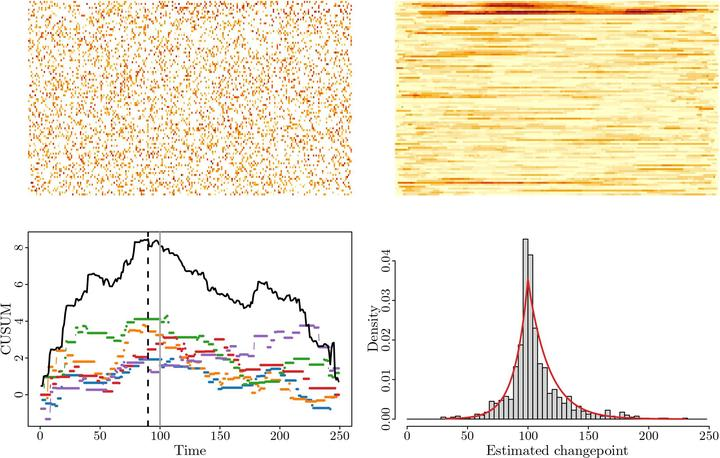High-dimensional changepoint estimation with heterogeneous missingnessAbstract

We propose a new method for changepoint estimation in partially-observed, high-dimensional time series that undergo a simultaneous change in mean in a sparse subset of coordinates. Our first methodological contribution is to introduce a ‘MissCUSUM’ transformation (a generalisation of the popular Cumulative Sum statistics), that captures the interaction between the signal strength and the level of missingness in each coordinate. In order to borrow strength across the coordinates, we propose to project these MissCUSUM statistics along a direction found as the solution to a penalised optimisation problem tailored to the specific sparsity structure. The changepoint can then be estimated as the location of the peak of the absolute value of the projected univariate series. In a model that allows different missingness probabilities in different component series, we identify that the key interaction between the missingness and the signal is a weighted sum of squares of the signal change in each coordinate, with weights given by the observation probabilities. More specifically, we prove that the angle between the estimated and oracle projection directions, as well as the changepoint location error, are controlled with high probability by the sum of two terms, both involving this weighted sum of squares, and representing the error incurred due to noise and the error due to missingness respectively. A lower bound confirms that our changepoint estimator, which we call ‘MissInspect’, is optimal up to a logarithmic factor. The striking effectiveness of the MissInspect methodology is further demonstrated both on simulated data, and on an oceanographic data set covering the Neogene period.

Type
Publication
arXiv 2108.01525